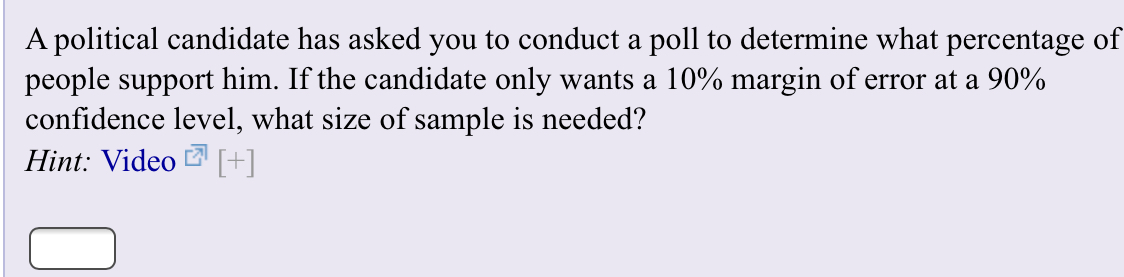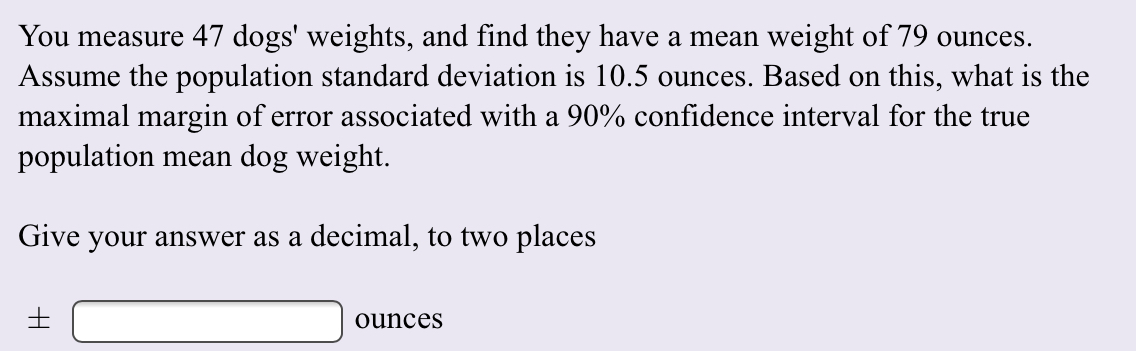# A political candidate has asked you to conduct a poll to determine what percentage ofpeople support him. If the candidate only wants a 10% margin of error at a 90%confidence level, what size of sample is needed?Hint: Video [] You measure 47 dogs' weights, and find they have a mean weight of 79 ounces.Assume the population standard deviation is 10.5 ounces. Based on this, what is themaximal margin of error associated with a 90% confidence interval for the truepopulation mean dog weight.Give your answer as a decimal, to two placesounces

Question
23 views

I need help finding the answer on the TI84 Plus CE and on paper ?. Thank youhelp_outlineImage TranscriptioncloseA political candidate has asked you to conduct a poll to determine what percentage of people support him. If the candidate only wants a 10% margin of error at a 90% confidence level, what size of sample is needed? Hint: Video [] fullscreenhelp_outlineImage TranscriptioncloseYou measure 47 dogs' weights, and find they have a mean weight of 79 ounces. Assume the population standard deviation is 10.5 ounces. Based on this, what is the maximal margin of error associated with a 90% confidence interval for the true population mean dog weight. Give your answer as a decimal, to two places ounces fullscreen
check_circle

Step 1

Note:

Hey there! Thank you for the question. As you have posted two different questions together, according to our policy, we have solved the first one for you. If you need help with the other question, please re-post it.

Step 2

Introduction:

The 100 (1 – α) % confidence interval for the population proportion, π is: (p – (/2­) (π (1 – π)/n), p + (/2­) (π (1 – π)/n)).

Here, n is the sample size, p is the sample proportion, and /2 is the critical value of the z-distribution, above which,...

### Want to see the full answer?

See Solution

#### Want to see this answer and more?

Solutions are written by subject experts who are available 24/7. Questions are typically answered within 1 hour.*

See Solution
*Response times may vary by subject and question.
Tagged in

### Other